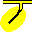#Luk

Luk is a programme for looking at the output matrices of Sap.

Luk is implemented in Fortran. It was originally developed under VMS, and later under Unix for Alpha, and is currently being developed under Debian GNU/Linux for Alpha and Intel, and under (alas) Microsoft Windows. The binaries can be made available.

There is no documentation beyond what you're now looking at.

## Usage

Luk takes as inputs various files produced by Sap. If the original model-definition file was `modelname.sap`, then either specify `modelname` on the command line or enter it in response to the `Model name?` prompt.

Luk will then check for the existence of the Sap output file `modelname.res`. If the file exists, Luk will read various parameters from it; if not, Luk will ask for them. The parameters are the number of equations; the bandwidth; the number of equations/block; and the number of load cases. Luk will then check for the existence of Sap's mass file, system-stiffness file, element-stiffness file, stress-transformation file and displacement file. Which analyses are available will depend on which files are available.

Luk will ask where to put its text output. The default filename extension is `.lst`. It will then ask which format to use when displaying matrix contents as text:

• E = standard Fortran E format
• M = quasi-metric format with metric suffixes
• Z = single character to indicate 0, + or -
• N = no output to file

Luk will then ask which analysis is desired. Respond by entering a unique abbreviation for one of the following:
 CONDITION Calc. condition number of system stiffness matrix 16CONDITION Calc. condition number using real*16 EIGEN Calc. eigenvalues/vectors of system RESID Calc. residuals for static problem MASS Look at system mass matrix DISP Look at displacements SYS-STIFF Look at system stiffness matrix EL-STIFF Look at element stiffness matrices STRESS-XFMN Look at element stress-xfmn matrices, etc. QUIT

The analysis of the system-stiffness matrix includes outputting the contents in the requested format; checking for parts of the model that are uncoupled from the rest; creating an image in which each pixel represents one element of the matrix and different colours are used to represent zero, positive and negative off-diagonal values; and determining the ranges for diagonal and off-diagonal elements.AudiLab home page
R. Funnell